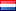### I need a rootfinder for a nonlinear fundtion in Matlab

Urgent
• Posted at : 1 year ago
• Post Similar Project
150

Budget
10
Proposals
169
Views
Awarded
Status
Skills Required

Posted By -

EVNetherlands
0.0
Projects Posted : 1
Projects Paid : 1
Services Purchased : 0
Total Spent :
30
Feedbacks : 0 %

#### Project Details hide (-)

I have written a function (file calculationdeterminant) which calculates a determinant that is function of k and omega. This function is complex. Now I need for each omega from 0 to 80 all the positive real roots (k's) of this function. For each omega there will be several roots. I need the values of the real roots where the function (real and imaginary part) is zero. I started by searching for the k's where the real part of the function is zero and then looked whether the imaginary part of the function is smaller than a certain tolerance, but I did not quite succeed in getting good results. Next to the values of the roots, I need a figure in which the roots are plotted with omega on the vertical axis and k on the horiontal axis. This can in first instance be plotted with dots, but ideally, next to the plot with dots I would need a figure (with omega on the vertical axis and k on the horizontal axis again) with smooth lines, comparable to the plot in attachment. Attached you find the Model data, the determinant function and an example of the plot I would need.